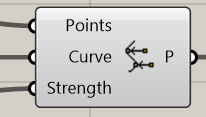# Kangaroo - Attract Points to a point

Using Kangaroo I can attract a point (or line segments) to a curve (OnCurve component), but …Can I use a Point as an attractor of another points? (not curve).
I would also like to have multiple attractor points, each one attracting its own Nearest Point.

I could of course make a micro size circle ( = curve) but I would prefer using points.

// Rolf

What would be the equivalent fot the K1-PowerLaw in K2 ?1 Like

If you want to pull a moving point towards a fixed target point, you can use the T input of the Anchor component.
If you want to pull one moving point towards another moving point you can use the ‘Coincident’ goal.

OK, that was a well hidden feature…But it works.

I have a Polyline (short segments, see grey dots) which I pull to a set of loops and I want the corner bends to be rounded with a minimum radius. However, using ClampAngle with vary small angle, I still get sharp corners on the pulled curve, see pic (the pipe is only for illustration of “should be”).

I don’t seem to use that component properly.

// Rolf

maxangle.gh (11.3 KB)
are you looking for something like this?

@DanielPiker, Yes, that’s pretty close to what I want. I reduced my strength’s and then got closer to your results.

However, I would like to have a “true maximium” (rigid maximum) angle between the segments. For example the following curve (tangent match to the straight lines at the ends) which I divided into 3 x 90 (270) segments with ClampAngle 1 degree. Then I’d expect at least 90 segments (1/3 of total segments) at each end of the curve to have max 1º angle. But it seems the Solver converges at much less segements than that (~40 seg’s in the pciture), meaning that it converged at +2º degree (although max was set to 1º).

It would have been interesting to be able to have a rigid minimum 279º between two segments (almost straight) and 180º (straight) where possible, that is, where minimum angle isn’t enforced by necessity. Or in other words, “default = straight aligned segment pairs, and bend only the minimum number of segments at each end to its limit angle”. (minimum and maximum notion swapped here, the current notion is a bit confusing…).

The real world problem which I’m exploring is trying to autofit some rubber hoses/pipes/wires while keeping tangency at the curve ends. For this I would need to stay within a limited bend radius as to not damage the pipe/hose/wire.

// Rolf

Hi @RIL, what would be the opposite to OnCurve, ie. using a curve or some points to ‘repel’ other points?

thank you Daniel!.
In this case, how is related the strength of the “Coincident” goal with the separation, the changing distance, between these 2 points?
I ask you this because with “PowerLaw” (K1) one can clearly deal with the distance.

2 Likes

Any news on how to recreate as close as possible the Plaw in K2? (force related to distance)

2 Likes

Power law seems functonal, unfortunately not in K2

Hi @Tom_F
There are several examples showing power law attraction and repulsion in Kangaroo2 in this thread:

5 Likes